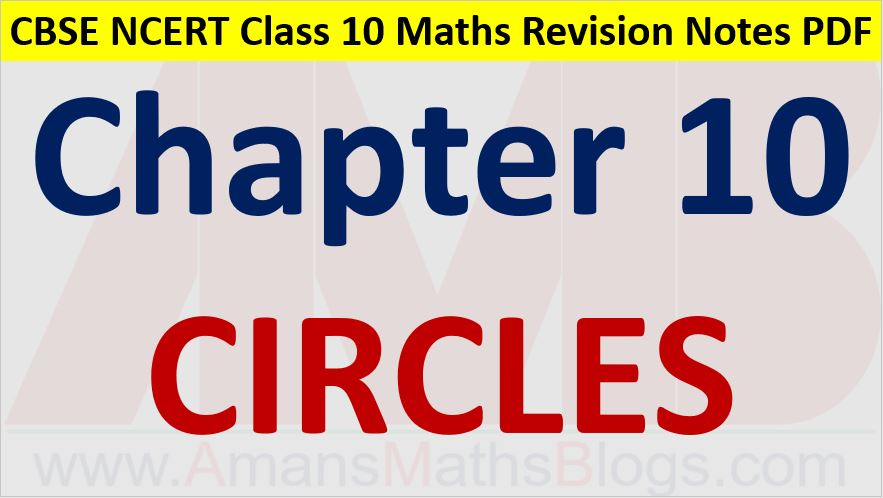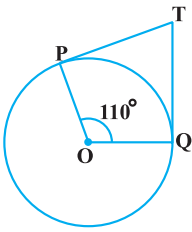Friday, October 22, 2021
Home > CBSE Class 10 > Circles CBSE NCERT Notes Class 10 Maths Chapter 10 PDF

# Circles CBSE NCERT Notes Class 10 Maths Chapter 10 PDFHi students, Welcome to AMBiPi (Amans Maths Blogs). In this article, you will get Circles CBSE NCERT Notes Class 10 Maths Chapter 10 PDF. You can download this PDF and save it in your mobile device or laptop etc.

# Circles CBSE NCERT Notes Class 10 Maths Chapter 10 PDF

## Circles

A circle is a collection of all points in a plane which are at a constant distance (radius) from a fixed point (centre).

## Tangent to Circles

A tangent to a circle is a line that intersects the circle at only one point.

The common point of the tangent and the circle is called the point of contact.

Thus, in the figure below, PQ is a tangent to circle and A is the point of contact. At any point (A) on a circle there can be one and only one tangent.## Tangent to Circles Theorems : 1

The tangent at any point of a circle is perpendicular to the radius through the point of contact.### Class 10 Maths Chapter 10 Examples 1:

A tangent PQ at a point P of a circle of radius 5 cm meets a line through the centre O at a point Q so that OQ = 12 cm. Find the length PQ.

Given that OQ = 12 and OP = 5

Using Pythagoras theorem, PQ = √(QO2 – OP2) = √(144 – 25) = √119

## Number of Tangent From a Point to Circles

(i) There is no tangent to a circle passing through a point lying inside the circle.

(ii) There is one and only one tangent to a circle passing through a point lying on the circle.

(iii) There are exactly two tangents to a circle through a point lying outside the circle.

The length of the segment of the tangent from the external point P and the point of contact with the circle is called the length of the tangent from the point P to the circle.## Tangent to Circles Theorems : 2

The lengths of tangents drawn from an external point to a circle are equal. (PQ = PR)

And, OP is the angle bisector of ∠QPR and ∠QOR.

And, ∠QPR + ∠QOR = 180o.### Class 10 Maths Chapter 10 Examples 2:

If TP and TQ are the two tangents to a circle with centre O so that ∠POQ = 110°, then find ∠PTQ.∠PTQ = 180 – 110 = 70o.

Click below to get CBSE Class 10 Maths Chapter wise Revision Notes PDF.

Chapter NoChapter name
Chapter 1 :Real Numbers CBSE Class 10 Maths Revision Notes
Chapter 2 :Polynomials CBSE Class 10 Maths Revision Notes
Chapter 3 :Pair of Linear Equations in Two Variables CBSE Class 10 Maths Revision Notes
Chapter 4 :Quadratic Equations CBSE Class 10 Maths Revision Notes
Chapter 5 :Arithmetic Progression CBSE Class 10 Maths Revision Notes
Chapter 6 :Triangles CBSE Class 10 Maths Revision Notes
Chapter 7 :Coordinate Geometry CBSE Class 10 Maths Revision Notes
Chapter 8 :Introduction to Trigonometry CBSE Class 10 Maths Revision Notes
Chapter 9 :Some Applications of Trigonometry CBSE Class 10 Maths Revision Notes
Chapter 10 :Circles CBSE Class 10 Maths Revision Notes
Chapter 11 :Constructions CBSE Class 10 Maths Revision Notes
Chapter 12 :Area Related to Circles CBSE Class 10 Maths Revision Notes
Chapter 13 :Surface Areas & Volumes CBSE Class 10 Maths Revision Notes
Chapter 14 :Statistics CBSE Class 10 Maths Revision Notes
Chapter 15 :Probability CBSE Class 10 Maths Revision Notes
AMBiPi
error: Content is protected !!